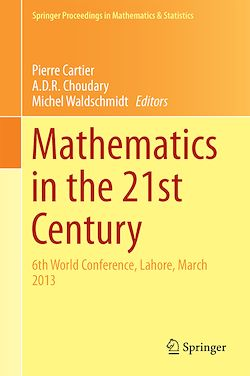# Mathematics in the 21st Century

-English
239 Pages

Description

Numerous well-presented and important papers from the conference are gathered in the proceedings for the purpose of pointing directions for useful future research in diverse areas of mathematics including algebraic geometry, analysis, commutative algebra, complex analysis, discrete mathematics, dynamical systems, number theory and topology. Several papers on computational and applied mathematics such as wavelet analysis, quantum mechanics, piecewise linear modeling, cosmological models of super symmetry, fluid dynamics, interpolation theory, optimization, ergodic theory and games theory are also presented.

Subjects

##### Discrete mathematics

Informations